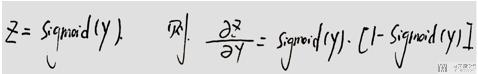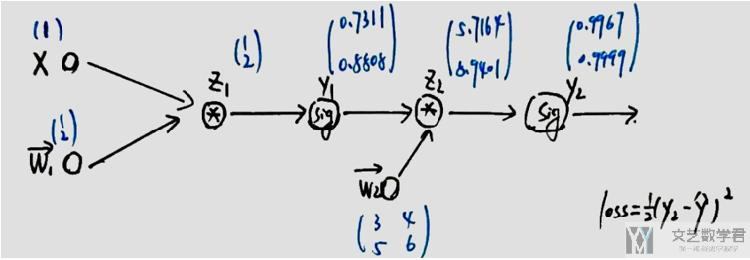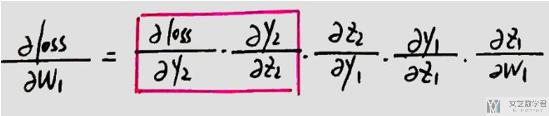# Pytorch入门教程03-正向传播与反向传播2020年10月3日07:39:00

2 1640字阅读5分28秒

## 简介

• 正向传播
• 反向传播

### Sigmoid函数导数## 正向传播

• 输入x=1
• 其中有权重参数w1和w2, 初始化如下图所示
• 整个运算过程为, y_pre = sigmoid(sigmoid(w1*x)*w2), loss = (y_pre-y_real)^21. def forwrad(x, y, w1, w2):
2.     # 其中 x,y 为输入数据，w为该函数所需要的参数
3.     z_1 = torch.mm(w1, x)
4.     y_1 = torch.sigmoid(z_1)
5.     z_2 = torch.mm(w2, y_1)
6.     y_2 = torch.sigmoid(z_2)
7.     loss = 1/2*(((y_2 - y)**2).sum())
8.     return loss, z_1, y_1, z_2, y_2

1. # 测试代码
2. x = torch.tensor([[1.0]])
3. y = torch.tensor([[1.0], [0.0]])
4. w1 = torch.tensor([[1.0], [2.0]], requires_grad=True)
5. w2 = torch.tensor([[3.0, 4.0], [5.0, 6.0]], requires_grad=True)
6. loss, z_1, y_1, z_2, y_2 = forwrad(x, y, w1, w2)
7. print(loss)
8. """
9. y1的值: tensor([[0.7311],
11. y2的值: tensor([[0.9967],
14. """

## 反向传播1. loss.backward()  # 反向传播，计算梯度
4. """
5. w1的梯度, tensor([[1.2243e-04],
6.         [7.8005e-05]])
7. w2的梯度, tensor([[-7.8431e-06, -9.4496e-06],
8.         [ 9.5752e-05,  1.1536e-04]])
9. """

• 微信公众号
• 关注微信公众号
•• QQ群
• 我们的QQ群号
•• 本文由 发表于 2020年10月3日07:39:00
• 转载请务必保留本文链接：https://mathpretty.com/12501.html